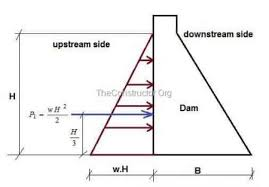## How to Calculate and Solve for Hydrostatic Pressure of a Dam | Water BudgetThe image above represents hydrostatic pressure of a dam.

To compute for hydrostatic pressure of a dam, two essential parameters are needed and these parameters are Specific Weight of Water (γ) and Depth of water (h).

The formula for calculating hydrostatic pressure of a dam:

Hn = γh² / 2

Where:

Hn = Hydrostatic Pressure of a Dam
γ = Specific Weight of Water
h = Depth of Water

Let’s solve an example;
Find the hydrostatic pressure of a dam when the specific weight of water is 10 and the depth of water is 5.

This implies that;

γ = Specific Weight of Water = 10
h = Depth of Water = 5

Hn = γh² / 2
Hn = (10)(5)² / 2
Hn = 10(25) / 2
Hn = 250 / 2
Hn = 125

Therefore, the hydrostatic pressure of the dam is 125.

Calculating the Specific Weight of Water when the Hydrostatic Pressure of the Dam and the Depth of Water is Given.

γ = Hn x 2 / h2

Where;

γ = Specific Weight of Water
Hn = Hydrostatic Pressure of a Dam
h = Depth of Water

Let’s solve an example;
Find the specific weight of water when the hydrostatic pressure of a dam is 30 and the depth of water is 6.

This implies that;

Hn = Hydrostatic Pressure of a Dam = 30
h = Depth of Water = 6

γ = Hn x 2 / h2
γ = 30 x 2 / 62
γ = 60 / 36
γ = 1.667

Therefore, the specific weight of water is 1.667.